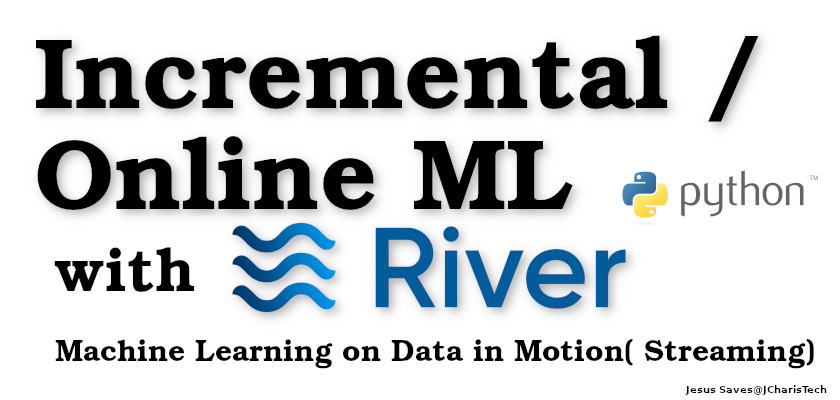# Online Machine Learning In Python with River (ML on Streaming Data)

The usual machine learning we perform is usually on data that is at rest, in other words we train our model on an already collected data which is in batches. This concept of building ML models on data at rest is termed Batch Machine Learning. It is the most common and useful.

However what if you want to perform ML on data that is in motion? What if you want to build ML models on streaming data? This concept of performing machine learning with data that is in motion is termed Online Machine Learning or Incremental Machine Learning.

By the end of this tutorial you will learn about

• Batch Learning Vs Online Machine Learning (Incremental ML)
• Libraries for Performing ML on Data In Motion
• How to Use River for Online ML
• How to build a text classifier with River
• How to check for model accuracy and classification report
• etc

The value and insight we can derive from data can be quite useful,however it is not all the time that you may get all the data at once or in batch or at rest. Therefore there is the need to find techniques to derive insight and perform ML on data that comes bit by bit (mini-batch) and data is incrementally coming one at a time.

These concepts described above refers to Batch Learning and Online Learning respectively.

So how do you perform ML on data that is coming one at a time? How do you build models on the go with data in motion? There are several libraries and framework to perform ML on data that is already at rest. Such tools include

• Scikit-learn
• Tensorflow
• PyTorch
• Caffe
• Mxnet
• etc.

For performing ML on data in motion or streaming data we can use tools such as

• Creme
• Scikit-Multiflow
• River (Combination of Creme + Scikit-Multiflow)
• SOA
• StreamDB (Spark Streaming)
• etc

Let us see how to use River in building ML models on data in motion .

### Using River For Online Machine Learning

River is A Python package for online/streaming machine learning. It caters for different ml problems, including regression, classification, and unsupervised learning. It can also be used for adhoc tasks, such as computing online metrics, and concept drift detection. It is a combined package consisting of Creme and Scikit-Multiflow

Installation

To install river you can use pip as below

`pip install river`

River like creme has a similar API like Scikit-learn and is also termed as the Scikit-learn for streaming or online machine learning. It supports almost all the different ML estimators and transformers but engineered for streaming data.

Let us see how to use river to build a simple text classifier model to classify text as either software or hardware. We will be using BagOfWords() as our transformer or vectorizer to convert our text into features and Naive Bayes MultinomialNB as our Machine Learning Estimator or Algorithm.

Let us import our packages

``````# Load ML Pkgs
from river.naive_bayes import MultinomialNB
from river.feature_extraction import BagOfWords,TFIDF

# Pipeline
from river.compose import Pipeline

# Metrics
from river.metrics import Classification_Report,Accuracy``````

Concerning the data used, in our case we will just use a list of tuples consisting of the text and the label(software or hardware). But you can also ingest data from a streaming engine or a csv file. However for a CSV File you will have to convert them to a dictionary or list of tuples in case you are working with the infamous Pandas package.

``````import pandas as pd
# Convert to Format
df.to_dict(records)

# Convert to Tuple
df.to_records(index=False)``````

Below is the data we will be using

``````data = [("my unit test failed","software"),
("tried the program, but it was buggy","software"),
("i need a new power supply","hardware"),
("the drive has a 2TB capacity","hardware"),
("unit-tests","software"),
("program","software"),
("power supply","hardware"),
("drive","hardware"),
("it needs more memory","hardware"),
("code","software"),
("API","software"),
("i found some bugs in the code","software"),
("i swapped the memory","hardware"),
("i tested the code","software")]
``````

Next we will build a pipeline consisting of two stages the transformer/vectorizer stage to convert our text to features and our estimator stage.

``````# Build Pipeline
pipe_nb = Pipeline(('vectorizer',BagOfWords(lowercase=True)),('nb',MultinomialNB()))

# Specify Steps
pipe_nb.steps

# Visualize Pipeline
pipe_nb.draw()
``````

Since the data is coming one at a time, we will have to fit our model on the data one at a time using the .learn_one(x,y) function of our pipeline during training. We can simulate this via iterating through our data using a for loop. [Notice that in creme t is .fit_one(x,y)]

``````# Train
for text,label in data:
pipe_nb = pipe_nb.learn_one(text,label)``````

#### Making Prediction

To make a prediction you can use the predict_one() function

``````# Make a Prediction
pipe_nb.predict_one('i built an API')

# Prediction Prob
pipe_nb.predict_proba_one('i built an API')
``````

#### Checking the Accuracy of the Model & Classification Report

In order to get the accuracy of our model and model performance you can use functions from the river.metrics sub-module such as Accuracy, Classification Report,etc

``````# Train
metric_acc = Accuracy()
for t,l in data:
y_pred_before = pipe_nb.predict_one(t)
# Check Acccuracy
metric_acc = metric_acc.update(l,y_pred_before)
pipe_nb = pipe_nb.fit_one(t,l)
#     print(metric_acc)

print("Final Accuracy",metric_acc)``````

To get the classification report we can use a test dataset and the Classification_Report function.

``````
samples = ["he writes code","the disk is faulty","no empty space on the drive"]
y_true = ["software","hardware","harware"]

y_pred = []
for i in samples:
res = pipe_nb.predict_one(i)
print("{}:Prediction:{}".format(i,res))
y_pred.append(res)

# Classification
report = ClassificationReport()
for yt, yp in zip(y_true, y_pred):
report = report.update(yt, yp)

# Get Report
report
``````

You have seen how to use River for online ML or incremental ML. You can also check out the video tutorial below.

### 2 thoughts on “Online Machine Learning In Python with River (ML on Streaming Data)”

1.1.